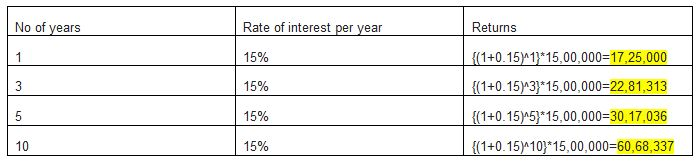mutual-fundlearn

# How are returns calculated in mutual funds when investing lumpsum amount?

When we invest in mutual funds, is the returns calculated on the invested amount or the returns are calculated on the total value (investment + returns)?

Ankit

Returns in case of lumpsum investments are calculated from the day of investment. In mutual funds, the returns is basically the difference between the selling price and the buying price.

Different ways of calculating returns are:

·     Absolute or Pint to Point Return- This technique helps the investors in calculating simple returns upon their initial investment. For calculating the same, the investors would need the initial as well as the ongoing or the last NAV (Net Asset Value) of the particular SIP scheme.

·     Simple Annualised Return- This technique is also known as the “effective annualized yield”. Formula used for calculating this is:

((1 + Absolute Rate of Return) ^ (365 / number of days)) -1.

·     Compounded Annual Growth Rate (CAGR)- depicts a figure that reveals the manner in which the investment should have grown if it had been generated as the steady return. But, in reality, the expected returns might not tend to be equal every year. This is the reason CAGR is used to represent the mean annual growth rate which tends to smoothen the volatility occurring in the returns upon a certain time period.

((( Ending Value / Beginning Value) ^ (1 / number of years)) – 1 * 100

When people are talking about returns, they are generally taking about annualised returns. XIRR is aggregation of multiple CAGRs which gives a better representation of the return being generated from mutual fund investments.

Ritika

While investing in lump sum amount, compounding is usually considered a better option for calculating returns since when one invests in lump sum the amount is usually higher and the returns are calculated on yearly basis.Consider for example one invests Rs. 15 Lakhs for 10 years for the average rate of return to be 15%. The amount returned is calculated as follows:

(1.15)^10 *15,00,000=INR 60.683 Lakhs

Consider year wise returns here as shown in the table: (here initial investment is considered to be 15 lakhs)And here we see that each year's returns are considered for next year's returns, which means power of compounding is applied here, since the principal amount for the second year is the one for the first year + returns on it, for third-year principal amount of the second year + the returns on it and so on..

the vice versa applies here if we need to find out returns percentage of the amount invested and the number of years given. Here is the formula:

CAGR= {(Ending value /Beginning value)^(1/n)}-1

Consider here the same example Ending value: 60.683Lakhs Beginning value=15 Lakhs; n=10 years. Applying the formula we get,

CAGR={(60.683/15)^(1/10)}-1=15% returns